## An automatic machine inserts mixed vegetables into a plastic bag. Past experience revealed that some packages were underweight and some were

Question

An automatic machine inserts mixed vegetables into a plastic bag. Past experience revealed that some packages were underweight and some were overweight, but most of them had satisfactory weight.
Weight % of Total Underweight 2.5 Satisfactory 90.0 Overweight 7.5a) What is the probability of selecting and finding that all three bags are overweight?b) What is the probability of selecting and finding that all three bags are satisfactory?

in progress 0
2 months 2021-08-06T14:17:17+00:00 1 Answers 0 views 0

a) 0.000016 = 0.0016% probability of selecting and finding that all three bags are overweight.

b) 0.729 = 72.9% probability of selecting and finding that all three bags are satisfactory

Step-by-step explanation:

The condition of the bags in the sample is independent of the other bags, which means that the binomial probability distribution is used to solve this question.

Binomial probability distribution

The binomial probability is the probability of exactly x successes on n repeated trials, and X can only have two outcomes.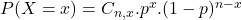In which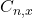is the number of different combinations of x objects from a set of n elements, given by the following formula.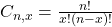And p is the probability of X happening.

a) What is the probability of selecting and finding that all three bags are overweight?

2.5% are overweight, which means that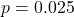3 bags means that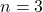This probability is P(X = 3). So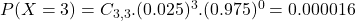0.000016 = 0.0016% probability of selecting and finding that all three bags are overweight.

b) What is the probability of selecting and finding that all three bags are satisfactory?

90% are satisfactory, which means that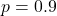3 bags means thatThis probability is P(X = 3). So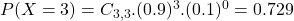0.729 = 72.9% probability of selecting and finding that all three bags are satisfactory